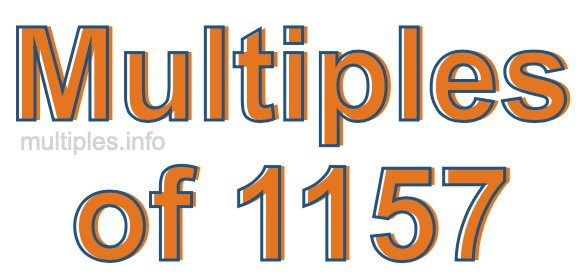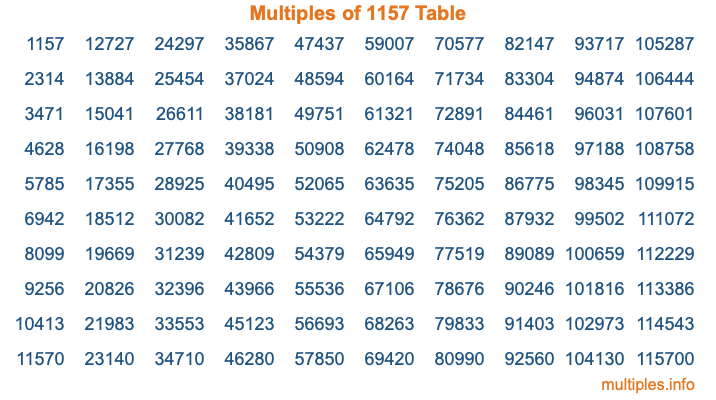Multiples of 1157Welcome to the Multiples of 1157 page. Here we will first teach you everything you will ever need to know about the multiples of 1157, and then give you a study guide summary of everything we taught you to make sure you remember it all. Use this page to look up facts and learn information about the multiples of 1157. This page will make you a multiples of one thousand one hundred fifty-seven expert!

Definition of Multiples of 1157
Multiples of 1157 are all the numbers that when divided by 1157 equal an integer. Each of the multiples of 1157 are called a multiple. A multiple of 1157 is created by multiplying 1157 by an integer.

Therefore, to create a list of multiples of 1157, you start with 1 multiplied by 1157, then 2 multiplied by 1157, then 3 multiplied by 1157, and so on for as long as you want. Thus, the list of the first five multiples of 1157 is 1157, 2314, 3471, 4628, and 5785. To see a larger list of multiples of 1157, see the printable image of Multiples of 1157 further down on this page. We also have a category where you can choose any nth multiple of 1157.

Multiples of 1157 Checker
The Multiples of 1157 Checker below checks to see if any number of your choice is a multiple of 1157. In other words, it checks to see if there is any number (integer) that when multiplied by 1157 will equal your number. To do that, we divide your number by 1157. If the the quotient is an integer, then your number is a multiple of 1157.

Is  a multiple of 1157?

Least Common Multiple of 1157 and ...
A Least Common Multiple (LCM) is the lowest multiple that two or more numbers have in common. This is also called the smallest common multiple or lowest common multiple and is useful to know when you are adding our subtracting fractions. Enter one or more numbers below (1157 is already entered) to find the LCM.

Check out our LCM Calculator if you need more details about the Least Common Multiple or if you need the LCM for different numbers for adding and subtraction fractions.

nth Multiple of 1157
As we stated above, 1157 is the first multiple of 1157, 2314 is the second multiple of 1157, 3471 is the third multiple of 1157, and so on. Enter a number below to find the nth multiple of 1157.

th multiple of 1157

Multiples of 1157 vs Factors of 1157
1157 is a multiple of 1157 and a factor of 1157, but that is where the similarities end. All postive multiples of 1157 are 1157 or greater than 1157. All positive factors of 1157 are 1157 or less than 1157.

Below is the beginning list of multiples of 1157 and the factors of 1157 so you can compare:

Multiples of 1157: 1157, 2314, 3471, 4628, 5785, etc.

Factors of 1157: 1, 13, 89, 1157

As you can see, the multiples of 1157 are all the numbers that you can divide by 1157 to get a whole number. The factors of 1157, on the other hand, are all the whole numbers that you can multiply by another whole number to get 1157.

It's also interesting to note that if a number (x) is a factor of 1157, then 1157 will also be a multiple of that number (x).

Multiples of 1157 vs Divisors of 1157
The divisors of 1157 are all the integers that 1157 can be divided by evenly. Below is a list of the divisors of 1157.

Divisors of 1157: 1, 13, 89, 1157

The interesting thing to note here is that if you take any multiple of 1157 and divide it by a divisor of 1157, you will see that the quotient is an integer.

Multiples of 1157 Table
Below is an image of the first 100 multiples of 1157 in a table. The table is in chronological order, column by column. The first column has the first ten multiples of 1157, the second column has the next ten multiples of 1157, and so on.The Multiples of 1157 Table is also referred to as the 1157 Times Table or Times Table of 1157. You are welcome to print out our table for your studies.

Negative Multiples of 1157
Although not often discussed or needed in math, it is worth mentioning that you can make a list of negative multiples of 1157 by multiplying 1157 by -1, then by -2, then by -3, and so on, to get the following list of negative multiples of 1157:

-1157, -2314, -3471, -4628, -5785, etc.

Multiples of 1157 Summary
Below is a summary of important Multiples of 1157 facts that we have discussed on this page. To retain the knowledge on this page, we recommend that you read through the summary and explain to yourself or a study partner why they hold true.

There are an infinite number of multiples of 1157.

A multiple of 1157 divided by 1157 will equal a whole number.

1157 divided by a factor of 1157 equals a divisor of 1157.

The nth multiple of 1157 is n times 1157.

The largest factor of 1157 is equal to the first positive multiple of 1157.

1157 is a multiple of every factor of 1157.

1157 is a multiple of 1157.

A multiple of 1157 divided by a divisor of 1157 equals an integer.

1157 divided by a divisor of 1157 equals a factor of 1157.

Any integer times 1157 will equal a multiple of 1157.

Multiples of a Number
Here you can get the multiples of another number, all with the same attention to detail as we did for multiples of 1157 on this page.

Multiples of
Multiples of 1158
Did you find our page about multiples of one thousand one hundred fifty-seven educational? Do you want more knowledge? Check out the multiples of the next number on our list!